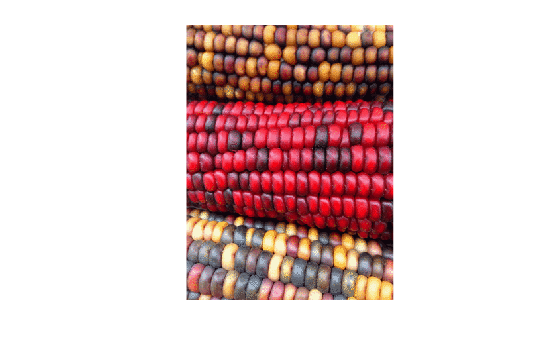ind2rgb

Convert indexed image to RGB image

Description

example

RGB = ind2rgb(X,map) converts the indexed image X and corresponding colormap map to RGB (truecolor) format.

Examples

collapse all

Read the first image in the sample indexed image file, corn.tif.

The indexed image X is a 415-by-312 array of type uint8. The colormap cmap is a 256-by-3 matrix of type double, therefore there are 256 colors in the indexed image. Display the image.

imshow(X,cmap)Convert the indexed image to an RGB image. The result is a 415-by-312-by-3 array of type double.

RGB = ind2rgb(X,cmap);

Check that values of the RGB image are in the range [0, 1].

disp(['Range of RGB image is [',num2str(min(RGB(:))),', ',num2str(max(RGB(:))),'].'])
Range of RGB image is [0.0078431, 0.97647].

Input Arguments

collapse all

Indexed image, specified as an m-by-n matrix of integers.

• If you specify X as an array of integer data type, then the value 0 corresponds to the first color in the colormap map. For a colormap containing c colors, values of image X are clipped to the range [0, c-1].

• If you specify X as an array of data type single or double, then the value 1 corresponds to the first color in the colormap. For a colormap containing c colors, values of image X are clipped to the range [1, c].

Data Types: single | double | uint8 | uint16

Colormap associated with indexed image X, specified as a c-by-3 matrix with values in the range [0, 1]. Each row of map is a three-element RGB triplet that specifies the red, green, and blue components of a single color of the colormap.

Data Types: double

Output Arguments

collapse all

RGB image, returned as an m-by-n-by-3 numeric array with values in the range [0, 1].

Data Types: double